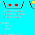Pages

Speed! - Number Sense - Factoring Algebraic Expressions

Speed! is a fun game for learning multiplication, but playing Speed! also helps children develop a strong number sense. This is the fourth post in a series of posts - Speed! Number Sense - to explore different mathmatical concepts and how playing Speed! can facilitate understanding of these concepts.

The fourth concept is FACTORING ALGEBRAIC EXPRESSIONS. When children play Speed! they begin to notice that some decks have the same numbers as other decks. That knowledge is an incredible aid in determining the least common denominator.

The first step is to factoring the equation is to find two numbers which multiply together to equal 28. Children who have played a lot of Four Speed and Seven Speed should recognize that both decks contain a 28.

The next step would be to check if the two numbers add to 11 to create the coefficient in front of the x. They do, and therefore the equation factors to.---------------------------------------------------------------------------------------------------------
Of course it is not always quite so simple.
This equation contains a 12. The first step is to find two numbers which multiply together to equal 12. Children who have played a lot of Speed should recognize that 12 is a card in several Speed! decks. In fact Two, Three, Four and Six Speed all contain the number 12.

The next step would be to find the combination of numbers which when multiplied together equal 12, and when added together equal 8.

The answer is 2 and 6, and therefore the equation can be factored as shown above.

Playing Speed! doesn't teach algebra, it is an activity that helps with multiplication and creates an excellent foundational number sense within children who play. It simply makes higher levels of math easier.

1 comment:

1.if students want to factoring or simplifying algebraic expression, they must need many cards, as we can see above, 12 has many factor 2, 3, 4 and 6.

Note: Only a member of this blog may post a comment.